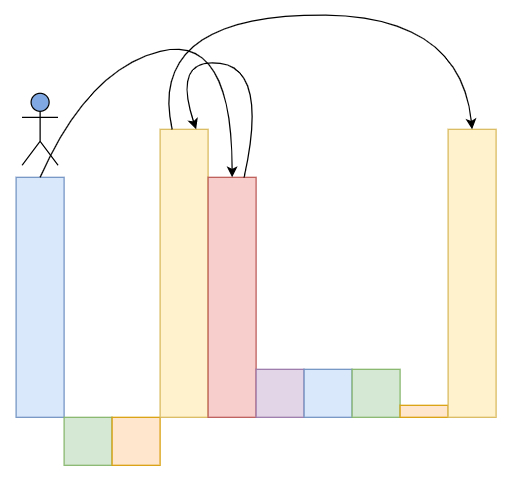# Jump Game IV in C++

Suppose we have an array of integers called arr. We are initially at index 0. In one step we can jump from index i to i + x where: i + x < n. i - x where: i - x >= 0. j where: arr[i] and arr[j] are same and i and j are not same. Here n is the size of array. We have to find the minimum number of steps to reach the last index of the array.

So, if the input is like,then the output will be 3, We need three jumps from index 0 to 4 to 3 to 9.

To solve this, we will follow these steps −

• Define one map m

• n := size of arr

• for initialize i := 0, when i < n, update (increase i by 1), do −

• insert i at the end of m[arr[i]]

• insert i at the end of m[arr[i]]

• insert 0 into visited

• Define one queue q

• for initialize lvl := 0, when not q is empty, update (increase lvl by 1), do−

• sz := size of q

• while sz is non-zero, decrease sz in each iteration by 1 do −

• curr := first element of q

• delete element from q

• if curr is same as n - 1, then

• return lvl

• i := curr

• if i - 1 >= 0 and not i - 1 is in visited, then −

• insert i - 1 into q

• insert i - 1 into visited

• if i + 1 < n and not i + 1 is in visited, then −

• insert i + 1 into q

• insert i + 1 into visited

• for initialize j := 0, when j < size of m[arr[curr]], update (increase j by 1), do −

• if (m[arr[curr], j]) is not in visited, then −

• insert m[arr[curr], j] into q

• insert m[arr[curr], j] into visited

• if arr[curr] is not in m, then −

• delete arr[curr] from m

• return -1

Let us see the following implementation to get better understanding −

## Example

Live Demo

#include <bits/stdc++.h>
using namespace std;
class Solution {
public:
int minJumps(vector<int>& arr) {
map<int, vector<int> > m;
int n = arr.size();
for (int i = 0; i < n; i++) {
m[arr[i]].push_back(i);
}
set<int> visited;
visited.insert(0);
queue<int> q;
q.push(0);
for (int lvl = 0; !q.empty(); lvl++) {
int sz = q.size();
while (sz--) {
int curr = q.front();
q.pop();
if (curr == n - 1)
return lvl;
int i = curr;
if (i - 1 >= 0 && !visited.count(i - 1)) {
q.push(i - 1);
visited.insert(i - 1);
}
if (i + 1 < n && !visited.count(i + 1)) {
q.push(i + 1);
visited.insert(i + 1);
}
for (int j = 0; j < m[arr[curr]].size(); j++) {
if (!visited.count(m[arr[curr]][j])) {
q.push(m[arr[curr]][j]);
visited.insert(m[arr[curr]][j]);
}
}
if (m.count(arr[curr])) {
m.erase(arr[curr]);
}
}
}
return -1;
}
};
main(){
Solution ob;
vector<int> v = {20,-5,-5,25,20,5,5,5,1,25};
cout << (ob.minJumps(v));
}

## Input

{20,-5,-5,25,20,5,5,5,1,25}

## Output

3